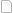• # Issue: TOC Nesting Issue

1.August 21, 2009 9:58 PM
pegasusVaultWiki Team
TOC Nesting Issue

When decreasing the H level by more than 1, the TOC nesting doesn't decrease by the appropriate amount.

Fixed for the next build by removing standard deviation and just tracking the open level numbers.Issue Details
Issue Number 793
Project VaultWiki 3.x Series
Status Fixed
Priority 4 - Warnings / Script Errors
Affected Version 2.5.1
Fixed Version 2.5.2
Milestone (none)
Software DependencyAny
Users able to reproduce bug 0
Users unable to reproduce bug 0
Assigned Users (none)Tags (none)1.August 22, 2009 4:52 AM
pegasusVaultWiki Team
After some users' requests, you can fix this yourself by making the following changes to vault/special_functions_postbit.php. Find:
Code:
`		\$done = array();`
Code:
`		\$zero = array();`
Find and delete:
Code:
```	if (\$depth > 7)
{
\$depth = 7;
}```
Find and delete:
Code:
`	\$zero["\$depth"] = \$depth;`
Find:
Code:
```		if (\$list["\$hix"]['level'] > \$zero["\$depth"])
{
if (\$list["\$hix"]['level'] < \$depth2)
{
\$depth2 = \$depth;
}
else if (\$hix == \$offset)
{
\$zero["\$depth"] = \$list["\$hix"]['level'];
\$depth2 = \$depth;
}
else if (\$list["\$hix"]['level'] == \$zero["\$depth"])
{
\$depth2 = \$depth;
}
else
{
\$depth2 = \$depth + 1;
}
}
else if (\$list["\$hix"]['level'] < \$zero["\$depth"])
{
\$depth2 = \$depth - 1;

if (\$depth2 < \$zero)
{
\$depth2 = \$zero;
}
}```
Replace with:
Code:
```		if (\$list["\$hix"]['level'] > \$last)
{
if (\$hix == \$offset)
{
\$zero[] = \$list["\$hix"]['level'];
\$last = \$list["\$hix"]['level'];
\$depth2 = \$depth;
}
else
{
\$depth2 = \$depth + 1;
}
}
else if (\$list["\$hix"]['level'] < \$last)
{
\$depth2 = \$depth - 1;
}```
Find and delete:
Code:
```		if (\$depth2 > 7)
{
\$depth2 = 7;
}```
Find:
Code:
`			\$tocbits .= construct_tocbits(\$list, \$hix, \$depth2, \$depthmark . intval(\$number - 1) . '.');`
`			array_pop(\$zero);`Reply
2.August 22, 2009 5:35 AM
StorytellerThanks a lot works fine now!Reply